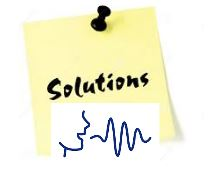NAHS AP Calculus Prerequisite Review

 Graphing, Trigonometry, Transcendental Functions Day Notes Critical Homework Videos A GraphingLesson A Critical HW SolutionsTranslating Functions Graphing Piece-wise Functions B TrigonometryLesson B Critical HW SolutionsThe Unit Circle Evaluating Trig. Functions Using the Unit Circle Evaluating Inverse Trig. Functions Solving Equations Containg Trig. Functions Graphing Trigonometric Functions C Graphing Calculator Lesson C Critical HW Solutions General Use of the TI 83/84 Finding Zeros of a Function Finding a Maximum or Minimum of a Function Finding Intersection Points Between Functions Graphing Points Using Lists D Exponential Function ReviewLesson D Critical HW SolutionsGraphing Exponential Functions Limits of Exponential Functions Applications of Exponential Functions Half-Life E Logarithmic Function ReviewLesson E Critical HW SolutionsGraphing Logarithmic Functions Limits of Logarithmic Functions Properties of Logarithms Solving Exponential Functions Using Logarithms Solving Equations Containing Logarithms